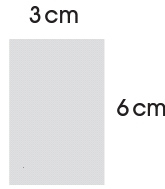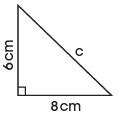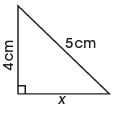# Second Term Examination Mathematics Primary 6 (Basic 6)

CRN’s INTERNATIONAL SCHOOL

SECOND TERM EXAMINATION

BASIC 6

SECTION A

### MATHEMATICS

Choose the correct answer from the options.

1. The length of a cloth is 1.85m. If 101 of such cloth are joined together, what be the total length?

[a] 2861m

[b] 28.18m

[c] 186.85m

2. The money paid by individuals to government is called ___________________.

[a] rate

[b] tax

[c] salary

3. Which of these is the same as 25?

[a] 2 x 25

[b] 2 x 5 x 2

[c] 5 x 5

4. How many seconds are there in 5 minutes?

[a] 300 secs

[b] 400 secs

[c] 40 secs

5. Which of these is the formula for finding the area of trapezium?

[a] L x b x h

[b] 1/2 ( a + b ) h

[c] 1/2 b x h

6. What is the perimeter of a rectangular tank which measures 25m by 12m?

[a] 37 m

[b] 74 m

[c] 84 m

Calculate the area of these shapes.

7.[a] 18 cm³

[b] 18 cm²

[c] 18 cm¹

8.[a] 10 cm¹

[c] 10 cm²

[c] 10 cm³

9. Pythagorean rule is used to solve problem on ___________________.

[a] circles

[b] right angle triangle

[a] straight lines

10. Given this Triangle, which of these is the pythagorean rule?[a] c² = a² + b²

[b] b³ = a² + c²

[c] c² = a² – b²

SECTION B

1a. Calculate the area of the following shapes:

(I)(II)2a. Use Pythagoras rule to find the length of the missing side ( ? ) in each right angle triangle:

(I)(II)b(I). A state government charges ₦2.50 as water rate for each taxable adult. How much will be collected from town with only 2500 taxable adults.

(II). How much will Ada for 1050 shares at 60 k share?

3a. A horse rider rides for 3 hours and covers a distance of 36 km. What is his average speed?

3b. An aeroplane flying at an average speed of 580km, spent 5 hours in the air. How many kilometers had been covered?

3c. If you brush your teeth for 2 minutes in the morning and 2 minutes in the evening, How much time will you spend brushing teeth in one year ?Give the time in hours and minute

(I). 24kg, 35kg, 52kg =

(II). 12t, 8400 kg, 39 t, 4900kg =

b. Convert 11 L to millilitres =

c. How many 4 L gallons are needed to fill a tank holding 112 L?

5a. Calculate the volume of a sphere with radius 7cm.

b. The radius of the base of a cylinder is 6cm and the height of the cylinder is 20cm. Calculate the:

(I). area of the base of the cylinder.

(II). volume of the cylinder.

c. The base of a prism 5cm high is right angled triangle. The sides containing the right angle are of the lengths 6cm and 9cm. Calculate the volume.

One Comment
1.